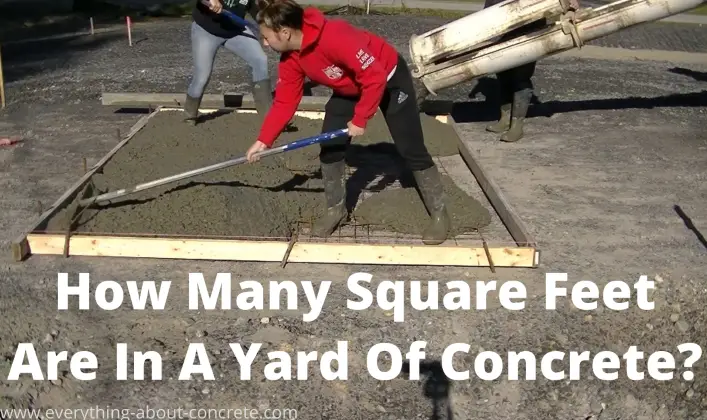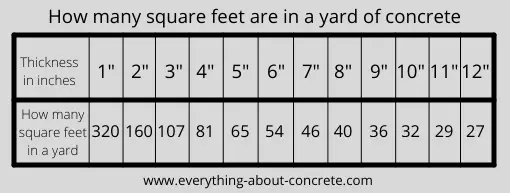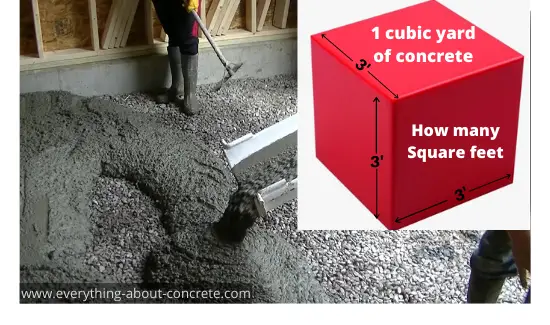# how many square feet are in a yard of concrete?IN ONE CUBIC YARD OF CONCRETE - there is 81 square feet at 4 inches thick.

The amount of square feet you'll get from a yard of concrete will be determined by how thick (depth) your concrete slab is.

A concrete slab that's 8' x 10' x 4" thick will take 1 yard of concrete.

How many square feet does a yard of concrete cover at different thicknesses?

The chart below shows you the exact amount of sq. ft. you'll get @ various depths.## how many square feet in a yard of concrete 4 inches thick?

Most of the concrete floors and slabs I do are 4 inches thick. That's a normal thickness for most garages, patios, walkways, and shed slabs.

If the concrete slab is 4 inches thick then I'll get 81 square feet from 1 yard of concrete.

• A 10' x 10' concrete slab that's 4" thick will take 1.23 yards of concrete
• A 10' x 12' concrete slab that's 4" thick will take 1.48 yards of concrete
• A 20' x 20' concrete slab that's 4" thick will take 4.94 yards of concrete
• A 24' x 24' concrete slab that's 4" thick will take 7.11 yards of concrete## how much concrete do you need?

You can use the chart above to figure how many yards of concrete you need.

Since the chart tells you how many square feet are in 1 yard of concrete at a certain depth, you can use this formula to figure total yardage for your project.

At 4" thick the formula would look like this:

• Length X Width ÷ 81 = yardage (example 24' x 24' = 576 sq. ft.  576 ÷ 81 = 7.11 yards of concrete)

At 6" thick the formula would look like this:

• Length X Width ÷ 54 = yardage (example 24' x 24' = 576 sq. ft.  576 ÷ 54 = 10.66 yards of concrete)

The number you divide by will be determined by the depth. Use the chart above to figure out which number that is for you based off the depth of your slab, patio, driveway, etc.

### use this concrete calculator to see how many yards you need

The concrete calculator below will help you determine the correct concrete yardage for your project.

Just type in your Length, Width, and thickness. Use feet and inches if you're in the USA, (or meter & centimeter) if you use the metric system.

## Slabs, Square Footings, or Walls

 length footinchyardmetercentimeter width footinchyardmetercentimeter thickness or height footinchyardmetercentimeter quantity

## Hole, Column, or Round Footings

 diameter footinchyardmetercentimeter depth or height footinchyardmetercentimeter quantity

## Circular Slab or Tube

 outer diameter footinchyardmetercentimeter inner diameter footinchyardmetercentimeter length or height footinchyardmetercentimeter quantity

#### how many bags of concrete makes a yard?

The amount of bags of concrete it takes to make a yard will depend on the size of the bag.

• It takes 45 bags of concrete to make a yard if the bag is 80 lbs.
• It takes 60 bags of concrete to make a yard if the bag is 60 lbs.
• It takes 75 bags of concrete to make a yard if the bag is 50 lbs.
• It takes 90 bags of concrete to make a yard if the bag is 40 lbs.

If you decide to use bags of Quikrete or Sakrete, find out how many bags of concrete are on a pallet.

#### how is a yard of concrete measured?

Concrete is measured in cubic yards. 1 cubic yard of concrete is 3 feet wide by 3 feet high by 3 feet deep. (3' x 3' x 3')

There are 27 cubic feet in one cubic yard. A cubic foot measures 12 inches wide x 12 inches high by 12 inches deep.

When you calculate yardage for your concrete slab, patio, floor, or driveway, you do it depending on how thick (depth) it is.

When you order ready-mix concrete that's delivered on a concrete truck, you order it by the cubic yard.

A bag of concrete is measured by the cubic foot. (because bags are small compared to a cubic yard)

• An 80 lb. bag of concrete will yield 0.60 cubic feet (or 1.8 square feet @ 4" thick)
• A 60 lb. bag of concrete will yield 0.45 cubic feet (or 1.35 square feet @ 4" thick)
• A 50 lb. bag of concrete will yield 0.37 cubic feet (or 1.11 square feet @ 4" thick)
• A 40 lb. bag of concrete will yield 0.30 cubic feet (or 0.90 square feet @ 4" thick)

One more way to share everything-about-concrete.com